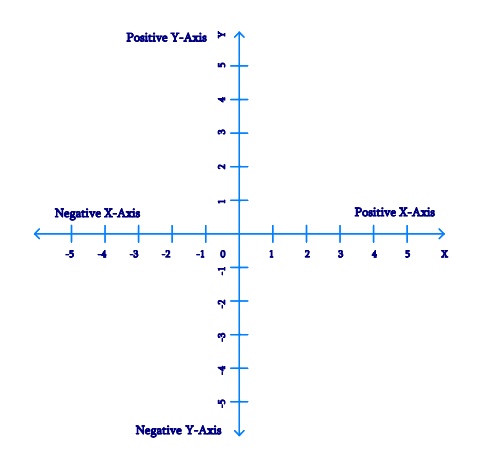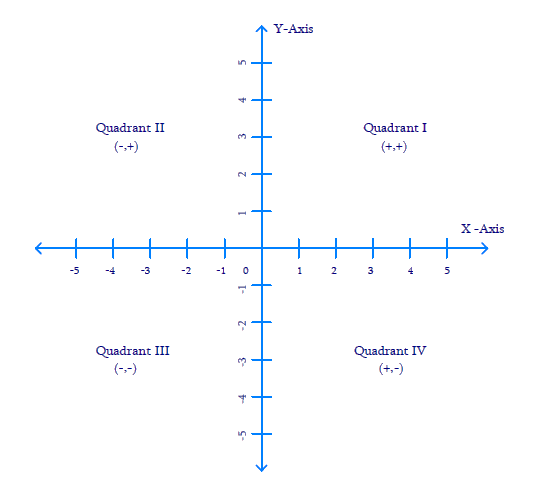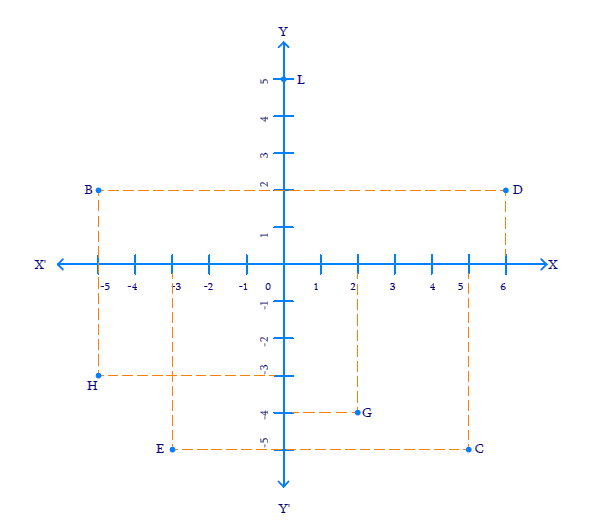# Exercise 3.2 Coordinate Geometry NCERT Solutions Class 9

## Chapter 3 Ex.3.2 Question 1

Write the answer of each of the following questions:

(i) What is the name of horizontal and the vertical lines drawn to determine the position of any point in the Cartesian plane?

(ii) What is the name of each part of the plane formed by these two lines?

(iii) Write the name of the point where these two lines intersect.

### Solution

Steps:

(i) The horizontal line that is drawn to determine the position of any point in the Cartesian plane is called as $$x-$$axis. The vertical line that is drawn to determine the position of any point in the Cartesian plane is called as $$y-$$axis.(ii) The name of each part of the plane that is formed by x-axis and y-axis is called as quadrant.(iii) The point, where the $$x-$$axis and the $$y-$$axis intersect is called as origin $$(O).$$

## Chapter 3 Ex.3.2 Question 2

See the given figure, and write the following:

(i)The coordinates of $$B.$$

(ii) The coordinates of $$C.$$

(iii) The point identified by the coordinates ($$–3, –5$$).

(iv) The point identified by the coordinates ($$2, –4$$).

(v) The abscissa of the point $$D.$$

(vi) The ordinate of the point $$H.$$

(vii) The coordinates of the point $$L.$$

(viii)The coordinates of the point $$M.$$### Solution

Steps:

From the Figure above,

(i) The co-ordinates of point $$B$$ is the distance of point $$B$$ from $$x-$$axis and $$y-$$axis. Therefore, the co-ordinates of point $$B$$ are ($$–5, 2$$).

(ii) The co-ordinates of point $$C$$ is the distance of point $$C$$ from $$x-$$axis and $$y-$$axis. Therefore, the co-ordinates of point $$C$$ are ($$5, –5$$).

(iii) The point that represents the co-ordinates ($$–3, –5$$) is $$E.$$

(iv) The point that represents the co-ordinates ($$2, –4$$) is $$G.$$

(v) The abscissa of point $$D$$ is the distance of point $$D$$ from the $$y-$$axis. Therefore, the abscissa of point $$D$$ is $$6.$$

(vi)  The ordinate of point $$H$$ is the distance of point $$H$$ from the $$x-$$axis. Therefore, the abscissa of point $$H$$ is $$–3.$$

(vii)  The co-ordinates of point $$L$$ in the above figure is the distance of point $$L$$ from $$x-$$axis and $$y-$$axis. Therefore, the co-ordinates of point $$L$$ are ($$0, 5$$).

(viii)  The co-ordinates of point $$M$$ in the above figure is the distance of point $$M$$ from $$x-$$axis and $$y-$$axis. Therefore, the co-ordinates of point $$M$$ are ($$–3, 0$$).

Instant doubt clearing with Cuemath Advanced Math Program